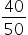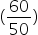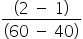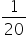Mathematics
Easy

Question

# There are 240 seniors in Kathryn’s school. Her class is planning a trip and is taking buses that hold a maximum of 50 passengers. Assume that the trip is optional.what is the average rate of change of the function over the interval from 40 to 60?

## 0.050.1101.25Hint:

## The correct answer is: 0.05

### x = 40 , f(x) = ceiling() = 1 ; (40 ,1)x = 60 , f(x) = ceiling= 2 ; (60 ,2)Rate of change (m) === 0.05.Hence, the correct option is B.

The ceiling function is also known as smallest integer function and the floor function is also known as the greatest integer function.

### Related Questions to study#### With Turito Foundation.#### Get an Expert Advice From Turito.